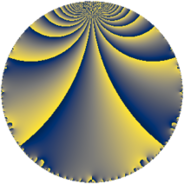# Properties

 Label 63.4.gLevel $63$ Weight $4$ Character orbit 63.g Rep. character $\chi_{63}(4,\cdot)$ Character field $\Q(\zeta_{3})$ Dimension $44$ Newform subspaces $1$ Sturm bound $32$ Trace bound $0$

# Related objects

## Defining parameters

 Level: $$N$$ $$=$$ $$63 = 3^{2} \cdot 7$$ Weight: $$k$$ $$=$$ $$4$$ Character orbit: $$[\chi]$$ $$=$$ 63.g (of order $$3$$ and degree $$2$$) Character conductor: $$\operatorname{cond}(\chi)$$ $$=$$ $$63$$ Character field: $$\Q(\zeta_{3})$$ Newform subspaces: $$1$$ Sturm bound: $$32$$ Trace bound: $$0$$

## Dimensions

The following table gives the dimensions of various subspaces of $$M_{4}(63, [\chi])$$.

Total New Old
Modular forms 52 52 0
Cusp forms 44 44 0
Eisenstein series 8 8 0

## Trace form

 $$44q + q^{2} - q^{3} - 79q^{4} + 38q^{5} - 20q^{6} - 7q^{7} - 24q^{8} - 31q^{9} + O(q^{10})$$ $$44q + q^{2} - q^{3} - 79q^{4} + 38q^{5} - 20q^{6} - 7q^{7} - 24q^{8} - 31q^{9} - 18q^{10} - 10q^{11} - 41q^{12} - 14q^{13} - 79q^{14} + 119q^{15} - 247q^{16} - 162q^{17} + 157q^{18} + 58q^{19} - 362q^{20} + 166q^{21} - 18q^{22} + 186q^{23} + 414q^{24} + 698q^{25} - 266q^{26} + 272q^{27} - 172q^{28} + 248q^{29} + 616q^{30} + 61q^{31} - 163q^{32} + 23q^{33} + 6q^{34} + 289q^{35} - 806q^{36} - 86q^{37} + 1522q^{38} - 565q^{39} + 36q^{40} - 692q^{41} + 395q^{42} - 86q^{43} - 443q^{44} - 1483q^{45} - 270q^{46} - 1005q^{47} - 1013q^{48} - 277q^{49} + 239q^{50} - 1719q^{51} + 670q^{52} + 258q^{53} + 910q^{54} - 870q^{55} + 714q^{56} + 566q^{57} - 474q^{58} - 1665q^{59} + 4q^{60} + 439q^{61} + 1812q^{62} + 493q^{63} + 872q^{64} - 613q^{65} + 3073q^{66} + 295q^{67} + 2748q^{68} + 1389q^{69} - 1044q^{70} + 636q^{71} + 981q^{72} - 338q^{73} - 2238q^{74} - 1064q^{75} + 1006q^{76} - 2909q^{77} + 157q^{78} + 133q^{79} - 4817q^{80} + 1325q^{81} + 6q^{82} - 1356q^{83} - 7081q^{84} + 483q^{85} + 6686q^{86} + 2774q^{87} - 738q^{88} - 2200q^{89} + 2665q^{90} + 1552q^{91} - 396q^{92} + 4365q^{93} - 1191q^{94} + 3083q^{95} - 1468q^{96} - 266q^{97} + 3601q^{98} - 5395q^{99} + O(q^{100})$$

## Decomposition of $$S_{4}^{\mathrm{new}}(63, [\chi])$$ into newform subspaces

Label Dim. $$A$$ Field CM Traces $q$-expansion
$$a_2$$ $$a_3$$ $$a_5$$ $$a_7$$
63.4.g.a $$44$$ $$3.717$$ None $$1$$ $$-1$$ $$38$$ $$-7$$